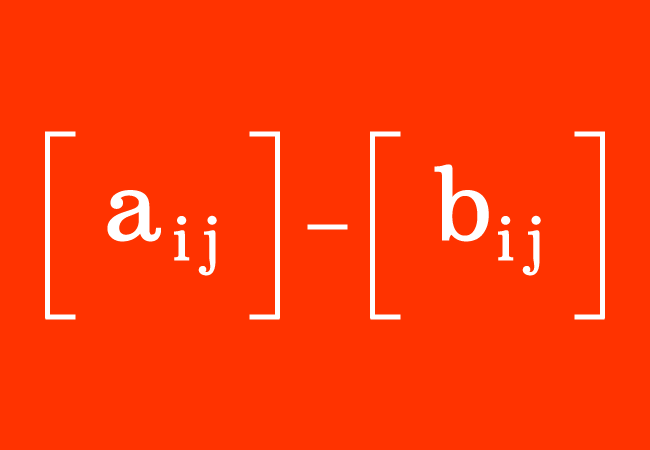# Matrix subtraction

A mathematical operation of subtracting a matrix from another matrix is called the subtraction of the matrices.

## IntroductionIn mathematics, the matrices often participate in the subtraction in some cases. Actually, every matrix consists of elements (or entries) in rows and columns. So, the matrices cannot be subtracted, like the subtraction of the numbers.

So, a systematic process is required for subtracting the matrices. Let’s learn how to subtract any two matrices in mathematics.

### Principle

Every element in a matrix is subtracted from the corresponding entry in another matrix is the principle for subtracting the matrices.

### Condition

The principle for subtracting the matrices can be applied for the matrices, which have the same order. In other words, it is possible to subtract the matrices if they have the same order.

$A$ $\,=\,$ $\begin{bmatrix} a_{11} & a_{12} & a_{13} & \cdots & a_{1n}\\ a_{21} & a_{22} & a_{23} & \cdots & a_{2n}\\ a_{31} & a_{32} & a_{33} & \cdots & a_{3n}\\ \vdots & \vdots & \vdots & \ddots & \vdots \\ a_{m1} & a_{m2} & a_{m3} & \cdots & a_{mn} \end{bmatrix}$ and $B$ $\,=\,$ $\begin{bmatrix} b_{11} & b_{12} & b_{13} & \cdots & b_{1n}\\ b_{21} & b_{22} & b_{23} & \cdots & b_{2n}\\ b_{31} & b_{32} & b_{33} & \cdots & b_{3n}\\ \vdots & \vdots & \vdots & \ddots & \vdots \\ b_{m1} & b_{m2} & b_{m3} & \cdots & b_{mn} \end{bmatrix}$

Let $A$ and $B$ be two matrices of the order $m \times n$. The arrangement of the elements in them is the same. So, it is possible to subtract an entry from the corresponding entry of another matrix.

$A-B$ $\,=\,$ $\begin{bmatrix} a_{11}-b_{11} & a_{12}-b_{12} & a_{13}-b_{13} & \cdots & a_{1n}-b_{1n}\\ a_{21}-b_{21} & a_{22}-b_{22} & a_{23}-b_{23} & \cdots & a_{2n}-b_{2n}\\ a_{31}-b_{31} & a_{32}-b_{32} & a_{33}-b_{33} & \cdots & a_{3n}-b_{3n}\\ \vdots & \vdots & \vdots & \ddots & \vdots \\ a_{m1}-b_{m1} & a_{m2}-b_{m2} & a_{m3}-b_{m3} & \cdots & a_{mn}-b_{mn} \end{bmatrix}$

The difference of the two matrices is also a matrix of the same order.

Remember that it is not possible to subtract the matrices of the different order due to the different arrangement of the elements in the matrices. Hence, it is impossible to subtract some entries and the subtraction is failed in this case.

### Examples

Let’s learn how to find the difference of the matrices from the following examples.

$(1).\,\,\,$ $\begin{bmatrix} 8 & 1 \\ 4 & 7 \\ \end{bmatrix}$ $-$ $\begin{bmatrix} 2 & 3 \\ 5 & 7 \\ \end{bmatrix}$

$\implies$ $\begin{bmatrix} 8 & 1 \\ 4 & 7 \\ \end{bmatrix}$ $-$ $\begin{bmatrix} 2 & 3 \\ 5 & 7 \\ \end{bmatrix}$ $\,=\,$ $\begin{bmatrix} 8-2 & 1-3 \\ 4-5 & 7-7 \\ \end{bmatrix}$

$\,\,\,\therefore\,\,\,\,\,\,$ $\begin{bmatrix} 8 & 1 \\ 4 & 7 \\ \end{bmatrix}$ $-$ $\begin{bmatrix} 2 & 3 \\ 5 & 7 \\ \end{bmatrix}$ $\,=\,$ $\begin{bmatrix} 6 & -2 \\ -1 & 0 \\ \end{bmatrix}$

Thus, the square matrices can be subtracted to find the difference of the matrices.

$(2).\,\,\,$ $\begin{bmatrix} 6 & 4 & 8 \\ 9 & 5 & 3 \\ \end{bmatrix}$ $-$ $\begin{bmatrix} 3 & 9 & 1 \\ 2 & 6 & 4 \\ \end{bmatrix}$

$\implies$ $\begin{bmatrix} 6 & 4 & 8 \\ 9 & 5 & 3 \\ \end{bmatrix}$ $-$ $\begin{bmatrix} 3 & 9 & 1 \\ 2 & 6 & 4 \\ \end{bmatrix}$ $\,=\,$ $\begin{bmatrix} 6-3 & 4-9 & 8-1 \\ 9-2 & 5-6 & 3-4 \\ \end{bmatrix}$

$\,\,\,\therefore\,\,\,\,\,\,$ $\begin{bmatrix} 6 & 4 & 8 \\ 9 & 5 & 3 \\ \end{bmatrix}$ $-$ $\begin{bmatrix} 3 & 9 & 1 \\ 2 & 6 & 4 \\ \end{bmatrix}$ $\,=\,$ $\begin{bmatrix} 3 & -5 & 7 \\ 7 & -1 & -1 \\ \end{bmatrix}$

In this way, the rectangular matrices can be subtracted for evaluating the difference of the matrices.

#### Problems

List of the questions on matrix subtraction with solutions for practice to learn how to subtract the matrices of the same order in mathematics.

Latest Math Topics
Jun 26, 2023
Jun 23, 2023

Latest Math Problems
Jul 01, 2023
Jun 25, 2023
###### Math Questions

The math problems with solutions to learn how to solve a problem.

Learn solutions

Practice now

###### Math Videos

The math videos tutorials with visual graphics to learn every concept.

Watch now

###### Subscribe us

Get the latest math updates from the Math Doubts by subscribing us.